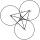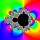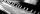# Triangle SSS

Calculate perimeter and area of ​​a triangle ABC, if a=40, b=36 and c=36.

Result

triangle perimeter:  112
area of triangle:  598.7

#### Solution:Try calculation via our triangle calculator.

Leave us a comment of example and its solution (i.e. if it is still somewhat unclear...):Be the first to comment!## Next similar examples:

1. TriangleCalculate heights of the triangle ABC if sides of the triangle are a=75, b=84 and c=33.
2. Triangle SAAThe triangle has one side long 71 m and its two internal angles is 60°. Calculate the perimeter and area of the triangle.
3. TriangleThe triangle has known all three sides: a=5.5 m, b=5.3 m, c= 7.8 m. Calculate area of ​this triangle.
4. Circles 2Calculate the area bounded by the circumscribed and inscribed circle in triangle with sides 12 cm, 14 cm, 18 cm.
5. Centre of massThe vertices of triangle ABC are from the line p distances 3 cm, 4 cm and 8 cm. Calculate distance from the center of gravity of the triangle to line p.
6. Three sidesSide b is 2 cm longer than side c, side a is 9 cm shorter than side b. The triangle circumference is 40 cm. Find the length of sides a, b, c . .. .
7. CirclesThree circles of radius 95 cm 78 cm and 64 cm is mutually tangent. What is the perimeter of the triangle whose vertices are centers of the circles?
8. TriangleDetermine whether a triangle can be formed with the given side lengths. If so, use Heron's formula to find the area of the triangle. a = 158 b = 185 c = 201
9. The farmerThe farmer would like to first seed his small field. The required amount depends on the seed area. Field has a triangular shape. The farmer had fenced field, so he knows the lengths of the sides: 119, 111 and 90 meters. Find a suitable way to determine th
10. SailsWe known heights 220, 165 and 132 of sail. It has triangular shape. What is the surface of the sail?
11. CalculationHow much is sum of square root of six and the square root of 225?
12. PowerNumber ?. Find the value of x.
13. Geometric progressionFill 4 numbers between 4 and -12500 to form geometric progression.
14. ProductThe product of two consecutive odd numbers is 8463. What are this numbers?Solve quadratic equation: 2x2-58x+396=0We want to prove the sentence: If the natural number n is divisible by six, then n is divisible by three. From what assumption we started?If Suzan practicing 10 minutes at Monday; every other day she wants to practice 2 times as much as the previous day, how many hours and minutes will have to practice on Friday?